## coremltools

Use Core ML to integrate machine learning models into your app. Core ML provides a unified representation for all models. Your app uses Core ML APIs and user data to make predictions, and to train or fine-tune models, all on the user’s device.

Core ML optimizes on-device performance by leveraging the CPU, GPU, and Neural Engine while minimizing its memory footprint and power consumption. Running a model strictly on the user’s device removes any need for a network connection, which helps keep the user’s data private and your app responsive.

# Convert a PyTorch Segmentation Model

This example demonstrates how to convert a PyTorch segmentation model to the Core ML format. The model takes an image and outputs a class prediction for each pixel of the image.

# Install the required software

Install the following:

pip install torch==1.6.0
pip install torchvision==0.7.0
pip install coremltools


# Load the model and image

To import code modules, load the segmentation model, and load the sample image, follow these steps:

1. Add the following import statements:
import urllib
import warnings
warnings.simplefilter(action='ignore', category=FutureWarning)

import torch
import torch.nn as nn
import torchvision
import json

from torchvision import transforms
from PIL import Image

import coremltools as ct

1. Load the DeepLabV3 model (deeplabv3) segmentation model:
model = torch.hub.load('pytorch/vision:v0.6.0', 'deeplabv3_resnet101', pretrained=True).eval()

1. Load the sample image:
input_image = Image.open("cat_dog.jpg")
input_image.show()Right-click and choose Save Image to download this test image.

# Normalize and segment the image

1. Apply normalization to the image using the PASCAL VOC mean and standard deviation values, which were applied to the model's training data. The following converts the image to a form that works with the segmentation model for testing the model's output.
preprocess = transforms.Compose([
transforms.ToTensor(),
transforms.Normalize(
mean=[0.485, 0.456, 0.406],
std=[0.229, 0.224, 0.225],
),
])

input_tensor = preprocess(input_image)
input_batch = input_tensor.unsqueeze(0)

1. Get predictions from the model. Running the normalized image through the model will compute a score for each object class per pixel, and the class will be assigned with a maximum score for each pixel.
with torch.no_grad():
output = model(input_batch)['out']
torch_predictions = output.argmax(0)

1. Plot the predictions, overlayed with the original image:
def display_segmentation(input_image, output_predictions):
# Create a color pallette, selecting a color for each class
palette = torch.tensor([2 ** 25 - 1, 2 ** 15 - 1, 2 ** 21 - 1])
colors = torch.as_tensor([i for i in range(21)])[:, None] * palette
colors = (colors % 255).numpy().astype("uint8")

# Plot the semantic segmentation predictions of 21 classes in each color
r = Image.fromarray(
output_predictions.byte().cpu().numpy()
).resize(input_image.size)
r.putpalette(colors)

# Overlay the segmentation mask on the original image
alpha_image = input_image.copy()
alpha_image.putalpha(255)
r = r.convert("RGBA")
r.putalpha(128)
seg_image = Image.alpha_composite(alpha_image, r)
seg_image.show()

display_segmentation(input_image, torch_predictions)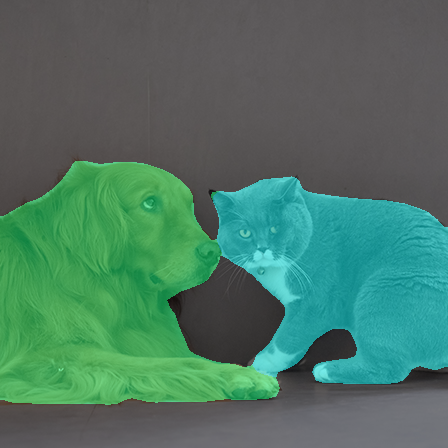# Trace the model with sample input

Now that the PyTorch model is segmenting the image correctly, you can trace the PyTorch model using the cat and dog image (cat_dog.jpg) as input. A random input of the same shape also works.

However, the model returns a dictionary. If you try to use trace = torch.jit.trace(model, input_batch) without first extracting the output you want from the dictionary, the tracer outputs an error: Only tensors or tuples of tensors can be output from traced functions.

To sidestep this limitation, you can wrap the model in a module that extracts the output from the dictionary:

class WrappedDeeplabv3Resnet101(nn.Module):

def __init__(self):
super(WrappedDeeplabv3Resnet101, self).__init__()
self.model = torch.hub.load(
'pytorch/vision:v0.6.0',
'deeplabv3_resnet101',
pretrained=True
).eval()

def forward(self, x):
res = self.model(x)
# Extract the tensor we want from the output dictionary
x = res["out"]
return x


Now the trace runs without errors:

traceable_model = WrappedDeeplabv3Resnet101().eval()
trace = torch.jit.trace(traceable_model, input_batch)


# Convert the model

Follow these steps:

1. Pass in the traced model to the Core ML converter, and include the inputs to provide to the model:
mlmodel = ct.convert(
trace,
inputs=[ct.TensorType(name="input", shape=input_batch.shape)],
)


### 📘Tip

This example includes a name for the output to make it easier to extract from the Core ML model's prediction dictionary. To learn more about input options, see Flexible Input Shapes.

1. Save the converted model:
mlmodel.save("SegmentationModel_no_metadata.mlmodel")


# Set the model's metadata

Set the model's metadata for previewing in Xcode, as described in Xcode Model Preview Types. Follow these steps:

1. Load the converted model from the previous step.
2. Set up the parameters. This example collects them in labels_json.
3. Define the model.preview.type metadata as "imageSegmenter".
4. Define the model.preview.parameters as labels_json.
5. Save the model.
# load the model
mlmodel = ct.models.MLModel("SegmentationModel_no_metadata.mlmodel")

labels_json = {"labels": ["background", "aeroplane", "bicycle", "bird", "board", "bottle", "bus", "car", "cat", "chair", "cow", "diningTable", "dog", "horse", "motorbike", "person", "pottedPlant", "sheep", "sofa", "train", "tvOrMonitor"]}

mlmodel.user_defined_metadata["com.apple.coreml.model.preview.type"] = "imageSegmenter"
mlmodel.user_defined_metadata['com.apple.coreml.model.preview.params'] = json.dumps(labels_json)

mlmodel.save("SegmentationModel_with_metadata.mlmodel")


# Open the model in Xcode

Double-click the saved SegmentationModel_with_metadata.mlmodel file in the Mac Finder to launch Xcode and open the model information pane: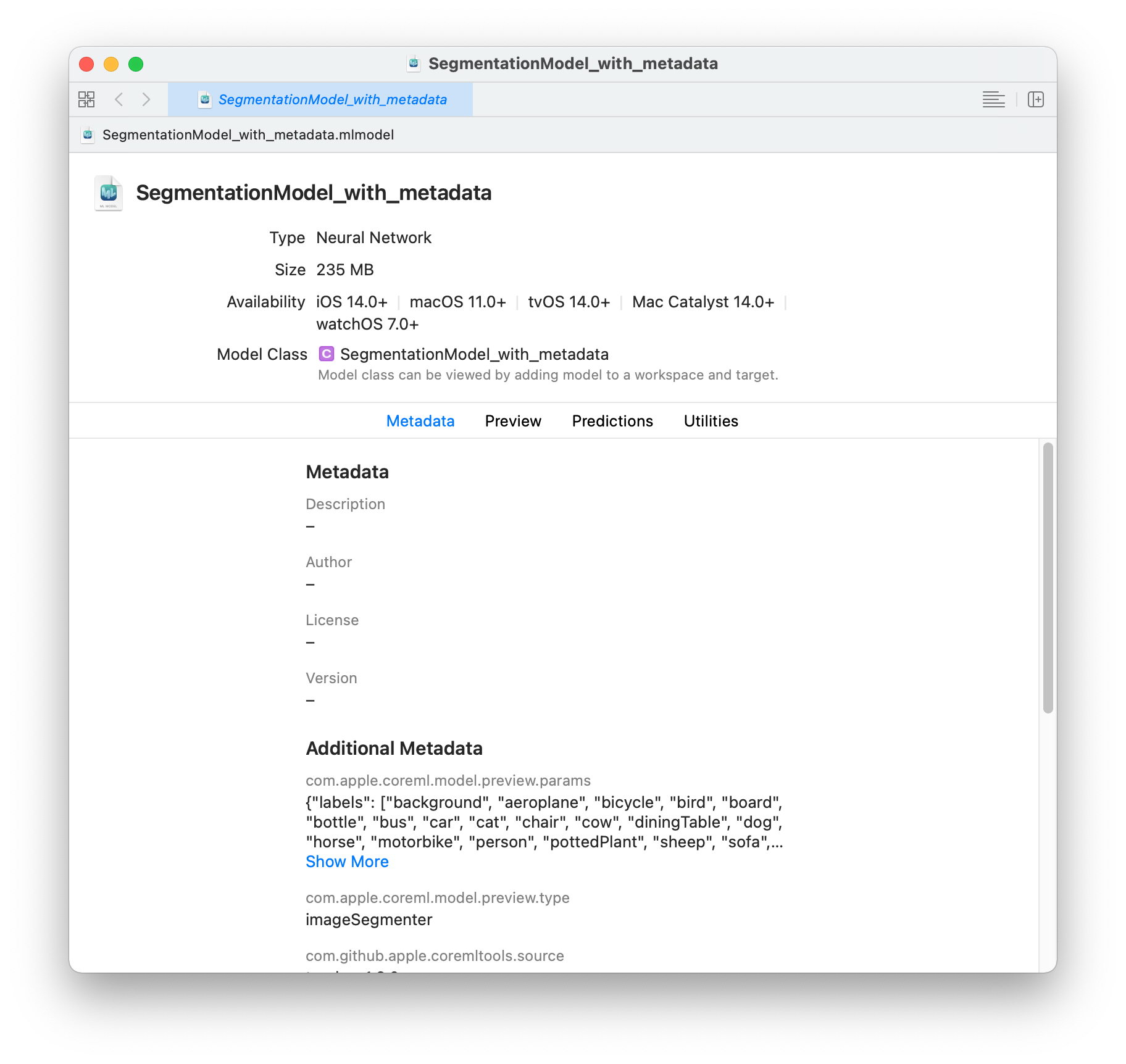The sample model offers tabs for Metadata, Preview, Predictions, and Utilities. Click the Predictions tab to see the model’s input and output.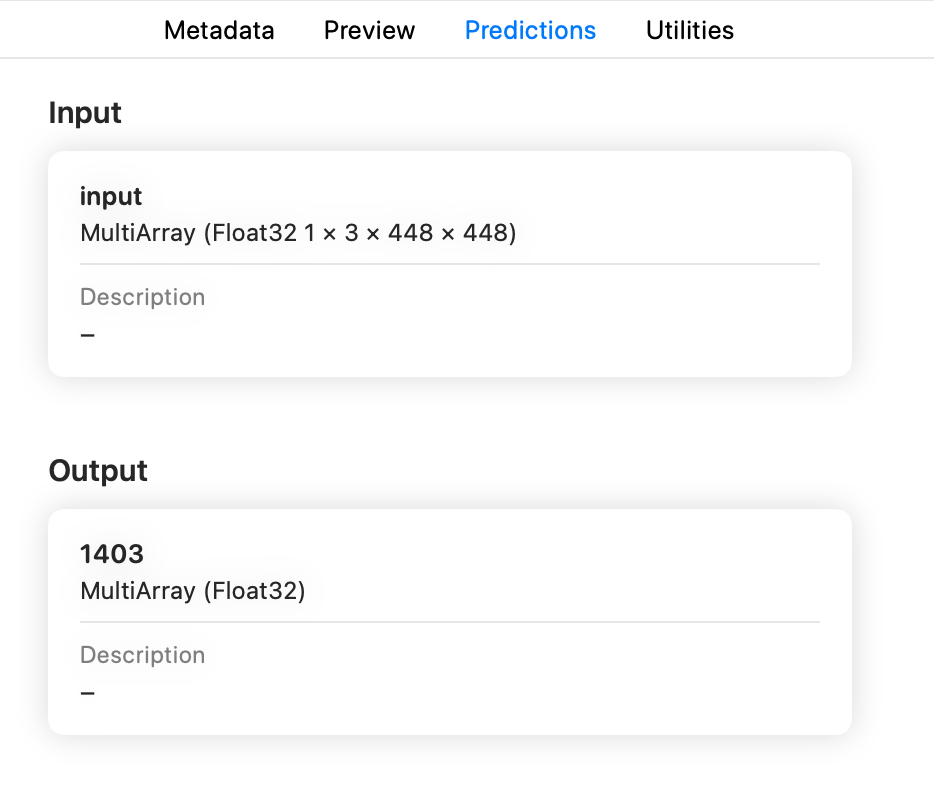### 📘Note

The preview for a segmentation model is available in Xcode 12.3 or newer.

To preview the model’s output for a given input, follow these steps:

1. Click the Preview tab.
2. Drag an image into the image well on the left side of the model preview. The result appears in the preview pane.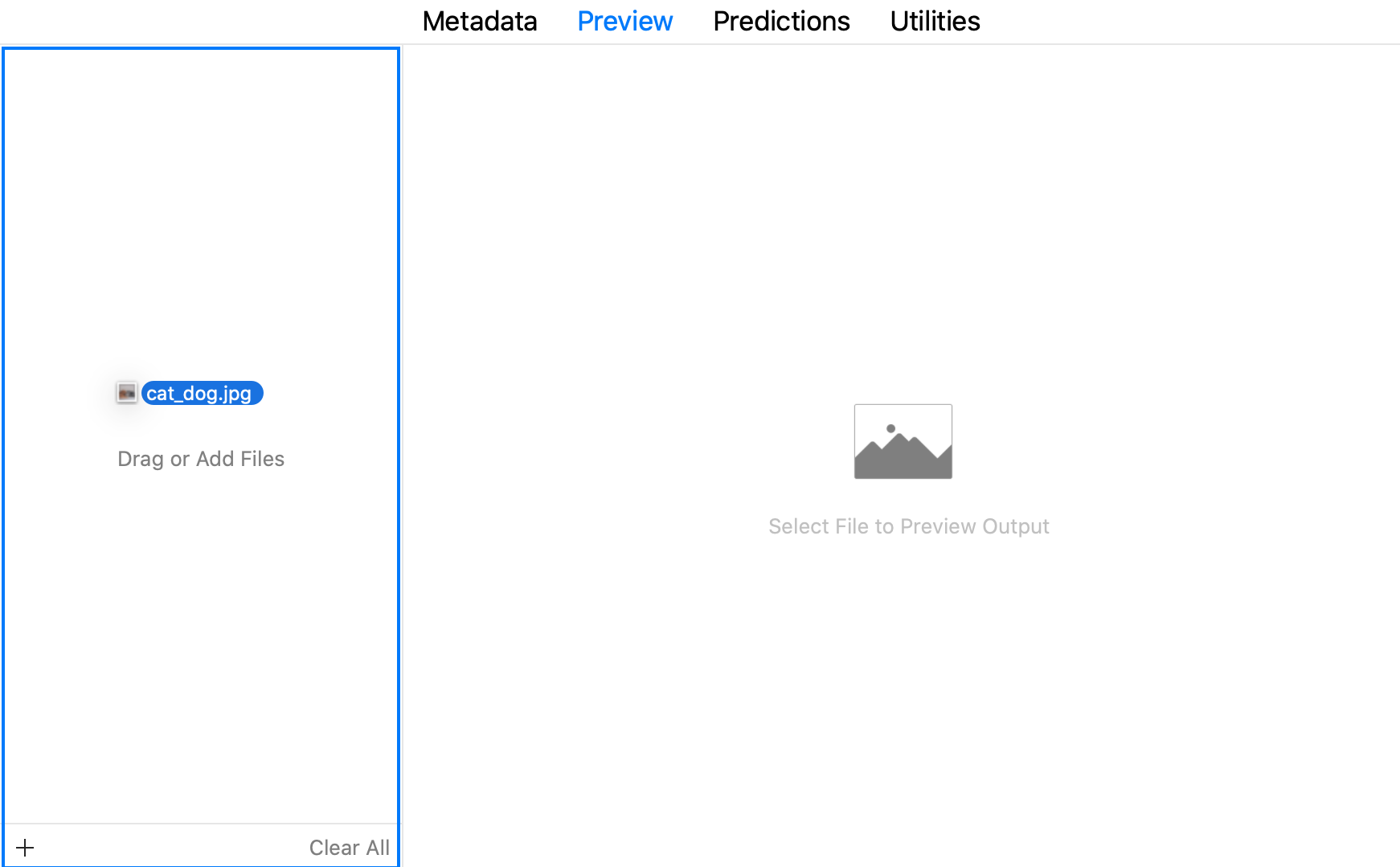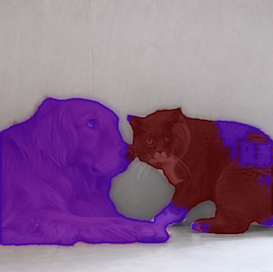### 📘Tip

To use the model with an Xcode project, drag the model file to the Xcode Project Navigator. Choose options if you like, and click Finish. You can then select the model in the Project Navigator to show the model information. For more information about using Xcode, see the Xcode documentation.

# Example code

The following is the full code for the segmentation model conversion.

Requirements:

pip install torch==1.6.0
pip install torchvision==0.7.0
pip install coremltools


Python code:

import urllib
import warnings
warnings.simplefilter(action='ignore', category=FutureWarning)

import torch
import torch.nn as nn
import torchvision
import json

from torchvision import transforms
from PIL import Image

import coremltools as ct

# Load the model (deeplabv3)
model = torch.hub.load('pytorch/vision:v0.6.0', 'deeplabv3_resnet101', pretrained=True).eval()

# Load a sample image (cat_dog.jpg)
input_image = Image.open("cat_dog.jpg")
input_image.show()

preprocess = transforms.Compose([
transforms.ToTensor(),
transforms.Normalize(
mean=[0.485, 0.456, 0.406],
std=[0.229, 0.224, 0.225],
),
])

input_tensor = preprocess(input_image)
input_batch = input_tensor.unsqueeze(0)

with torch.no_grad():
output = model(input_batch)['out']
torch_predictions = output.argmax(0)

def display_segmentation(input_image, output_predictions):
# Create a color pallette, selecting a color for each class
palette = torch.tensor([2 ** 25 - 1, 2 ** 15 - 1, 2 ** 21 - 1])
colors = torch.as_tensor([i for i in range(21)])[:, None] * palette
colors = (colors % 255).numpy().astype("uint8")

# Plot the semantic segmentation predictions of 21 classes in each color
r = Image.fromarray(
output_predictions.byte().cpu().numpy()
).resize(input_image.size)
r.putpalette(colors)

# Overlay the segmentation mask on the original image
alpha_image = input_image.copy()
alpha_image.putalpha(255)
r = r.convert("RGBA")
r.putalpha(128)
seg_image = Image.alpha_composite(alpha_image, r)
# display(seg_image) -- doesn't work
seg_image.show()

display_segmentation(input_image, torch_predictions)

# Wrap the Model to Allow Tracing*
class WrappedDeeplabv3Resnet101(nn.Module):

def __init__(self):
super(WrappedDeeplabv3Resnet101, self).__init__()
self.model = torch.hub.load('pytorch/vision:v0.6.0', 'deeplabv3_resnet101', pretrained=True).eval()

def forward(self, x):
res = self.model(x)
x = res["out"]
return x

# Trace the Wrapped Model
traceable_model = WrappedDeeplabv3Resnet101().eval()
trace = torch.jit.trace(traceable_model, input_batch)

# Convert the model
mlmodel = ct.convert(
trace,
inputs=[ct.TensorType(name="input", shape=input_batch.shape)],
)

# Save the model without new metadata
mlmodel.save("SegmentationModel_no_metadata.mlmodel")

# Load the saved model
mlmodel = ct.models.MLModel("SegmentationModel_no_metadata.mlmodel")

# Add new metadata for preview in Xcode
labels_json = {"labels": ["background", "aeroplane", "bicycle", "bird", "board", "bottle", "bus", "car", "cat", "chair", "cow", "diningTable", "dog", "horse", "motorbike", "person", "pottedPlant", "sheep", "sofa", "train", "tvOrMonitor"]}

mlmodel.user_defined_metadata["com.apple.coreml.model.preview.type"] = "imageSegmenter"
mlmodel.user_defined_metadata['com.apple.coreml.model.preview.params'] = json.dumps(labels_json)

mlmodel.save("SegmentationModel_with_metadata.mlmodel")Updated 4 months ago

# Convert a PyTorch Segmentation Model

### Suggested Edits are limited on API Reference Pages

You can only suggest edits to Markdown body content, but not to the API spec.